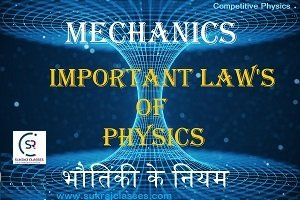# Law’s of Physics (Mechanics)भौतिकी के नियम

Physics topic- “Law’s of Physics (Mechanics)-भौतिकी के नियम” is important for all competitive exams like CET- Common eligibility Test, SSC CGL, SSC CHSL, RRB NTPC, UPSC and other state civil services exams. In these exams, almost 4-5 questions are coming from Physics. Let’s start the topic- Law’s of Physics (Mechanics)-भौतिकी के नियम.

## (भौतिकी के नियम)

Laws of science are statements, based on repeated experiments or observations that describe or predict a range of natural phenomena. Laws are developed from data and can be further developed through mathematics in all cases they are directly or indirectly based on empirical evidence.

• Scientific law describes what happens, whereas scientific theories explain why something happens.

Newton’s laws of motion:

Newton’s laws of motion states relations between the forces acting on a body and the motion of the body. Newton’s laws of motion were given by “Sir Isaac Newton”.

Newton’s First Law of Motion:

Newton’s first law states that an object remains in the state of rest or in a uniform state of motion unless an external force acts on it.

In simple words we can say that there must applied an external force for any change in velocity (a change in either magnitude or direction) to change the state of object. It is also called Law of Inertia.

External forces are friction, normal force and air resistance.

Examples of newton’s first law of motion: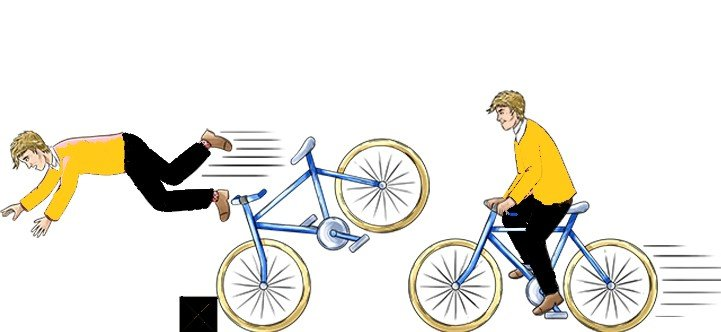• When a vehicle starts from rest, the person setting inside fell backward jerk. While a sudden brake applied to the vehicle, person bands ahead, because inertia (the tendency to remain unchanged) tries to keep the passenger in the same state.
• The first law explains why you go falling over the handlebars if your bike stops suddenly.
• Dust get remove from carpet on beating it.
• Water gets remove from cloths when we jerk wet cloths, .
• When we shake branch of a tree, fruits drops from it.
• At the time of boding and de – boding person runs towards the direction of vehicle.
• When a ball thrown above from a moving vehicle, it return back in hand of the thrower.

Newton’s Second Law of Motion :

The net or resultant force acting on an object is equal to the rate of change of momentum. For a constant mass, force equals mass times acceleration.

• In mathematical form it is written as F = ma

Where, F is force, ‘m’ is mass and ‘a’ is acceleration.

Newton’s second law states that the acceleration of an object depends upon two variables – the net force acting on the object and the mass of the object.  The acceleration of the body is directly proportional to the net force acting on the body and inversely proportional to the mass of the body.

• Due to this there are two conditions occurs:
1. If the force acting upon an object is increased, then the acceleration of the object is increased.
2. If the mass of an object is increased, then the acceleration of the object is decreased.

Examples of Newton’s 2nd law of motion: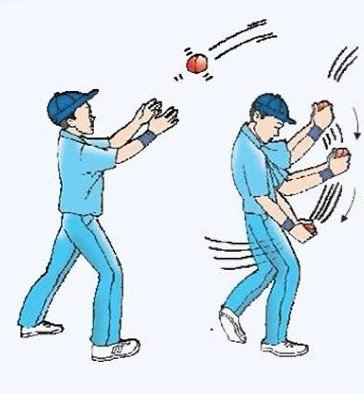• When a fielder catches the ball, he moves his hands in the direction of the ball, to reduce the hardness.
• At the place of high-jump/long-jump either land dig or mattress placed to reduce the hardness.
• Working of “shocker” in vehicles.
• Thermocols are placed in between the glass, when carrying them one place to other.
• Air balloon open in vehicles at the time of accident and it do help save the person life.

Newton’s Third Law of motion:

It state that “for every action in nature there is an equal and opposite reaction”.

When one body exerts a force on a second body, the second body simultaneously exerts a force in equal magnitude and opposite direction on the first body.

This forces is also called action and reaction forces.

Examples of newton’s third law:• Walking/ Running of a man.
• Firing by bullet.
• Throw darts (shoot arrows).
• Sailing of boat.
• At the time of pull out of water from the well. If rope breakdown then person will through opposite from the well.

Law’s of Physics (Mechanics)-भौतिकी के नियम

Archimedes’ principle:

It states that when a body immersed in a fluid, (whether partially or fully submerged) it experiences a buoyant force equal to the weight of the fluid that the body displaced and acts in the upward direction at the centre of mass of the displaced fluid”.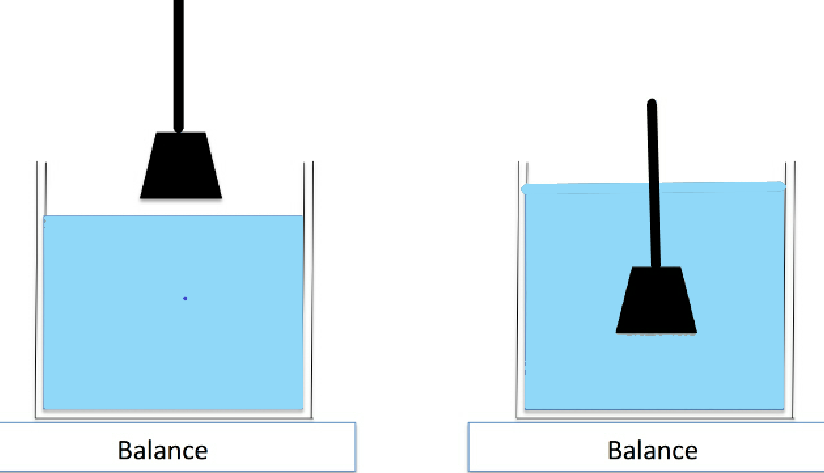• It is also known as “law of Buoyancy”

Applications of Archimedes principle:

• Submarine.
• Hot-air balloon
• Hydrometer.

Bernoulli’s Principle:

Bernoulli’s Principle states that as the speed of a moving fluid (liquid or gas) increases, the pressure within the fluid decreases.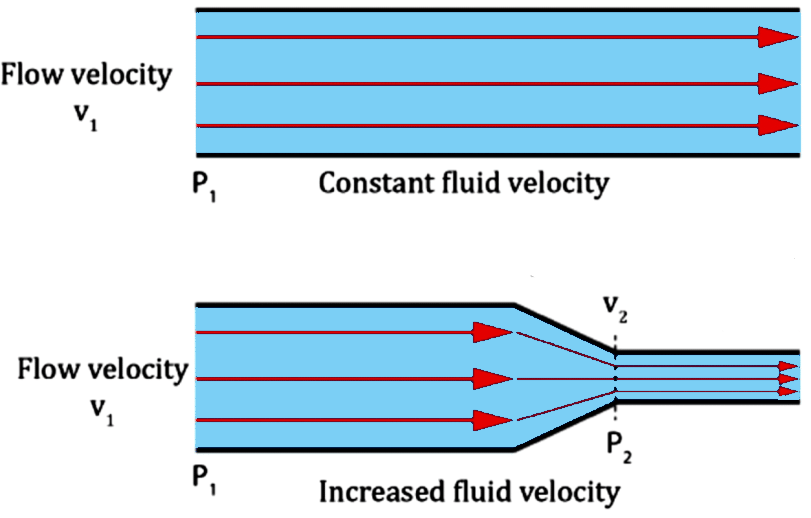Application of Bernoulli’s Principle:

• Sprayer works on the Bernoulli’s principle.
• During the arrival of train at platform, we put some steps behind from platform to make safe to self, otherwise we fall down on railway track according to Bernoulli’s theorem.
• The aerodynamic lift on the wing of an Aeroplane is also explained in part by this principle.
• At the time of storm, the roof of hut blown up.

Law’s of Physics (Mechanics)-भौतिकी के नियम

Pascal’s law/Pascal’s principle:-

Pascal’s law states that in a fluid at rest in a closed container, a pressure change in one part is transmitted without loss to every portion of the fluid and to the walls of the container.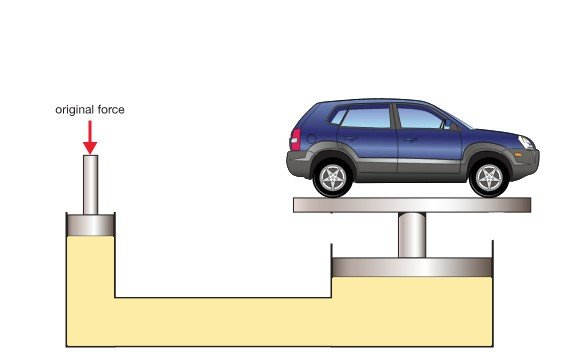• This principle was given by the French scientist Blaise Pascal.
• The SI unit of pressure is “pascal”.

Application of pascal’s law:

• Hydraulic breaks.
• Power breaks
• Door power close shockers
• Power staring.

Ohm’s Law:

Ohm’s law states that the voltage across a conductor is directly proportional to the current flowing through it, at provided all physical conditions and temperature remain constant.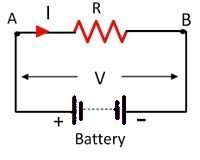It can be written as:  V= IR

Where, as V is voltage, I is current, R is resistance.

Ohm’s law is also used in dc ammeter and other dc shunt motors to divert the current.

Snell’s law:

The refraction of light when it passes from a fast medium to a slow medium bends the light ray toward the normal to the boundary between the two media. The amount of bending depends on the indices of refraction of the two media and is described quantitatively by Snell’s Law.

Snell’s law states that the ratio of the sines of the angles of incidence and refraction is equivalent to the ratio of phase velocities in the two media, or equivalent to the reciprocal of the ratio of the indices of refraction.

Formula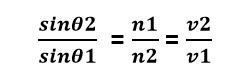Where, θ angle measured from the normal of the boundary

v = velocity of light

n = refractive index

Law’s of Physics (Mechanics)-भौतिकी के नियम

Capillary action: –

Capillary action is the ability of a liquid to flow in narrow spaces.

Application of capillary action: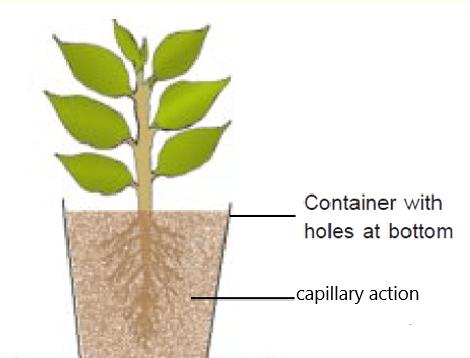• Drawing up of liquids between the hairs of a paint-brush
• In a thin tube
• In porous materials such as paper and plaster.
• The oil rise in wick due to capillary action.
• Water observed by towel from body, due to capillary action.
• Oil spread in hairs due to capillary action.
• Water spread in field, due to capillary action.
• Water rise in plant, due to capillary action and other actions also take place in this process are osmosis and transpiration.

Density:

Density is defined as the ratio of an object’s mass to its volume

• The formula for density (d) is =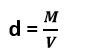Where, d is density, M is mass, and V is volume.

• Swimming in ocean is easier than river because density of ocean water is more than river.
• An iron ball float in mercury because density of mercury more than iron.
• Ice float on the water, because water’s density is more than ice.
• When a ship enters from river to sea their level increase due to centre of gravity and density.
• If water is boiled, floating wooden clot start to emerging in water.

Law’s of Physics (Mechanics)-भौतिकी के नियम

Centre of gravity:

The centre of gravity defined as the density is same throughout the object.

Facts of Centre of Gravity: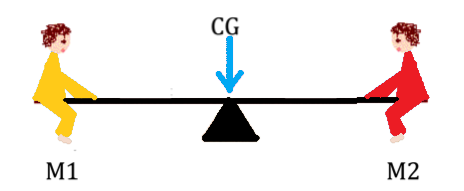• The centre of gravity is the average location of the weight of an object.
• In less amount of water, the ship became sink due to high centre of gravity.
• All luxury and racing car are low floor due to high centre of gravity.
• The bottom of ship is made up of heavy material due to maintaining high centre of gravity.
• Bridge are made up of Dom shaped to reduce the centre of gravity.

Surface tension (Facts):-

Surface tension is known “as A tendency of liquid surfaces to shrink into the minimum surface area possible”. It happens due to the cohesive nature of its molecules.• The rain drops become spherical due to “surface tension.”
• All heavily bodies becomes spherical due to reduce “surface tension”.
• Foam of the soap clean the cloths by reducing the surface tension of the water.
• Oil poured in cooler water, the larvae of mosquito sink to die by reducing the surface tension of water.

This law states that total number of atoms/molecules is directly proportional to the volume occupied by the gas at constant temperature and pressure.

• “Equal volumes of all gases, at the same temperature and pressure, have the same number of molecules.”
• Note : For a given mass of an ideal gas, the volume and amount (moles) of the gas are directly proportional if the temperature and pressure are constant.

Example:

Equal volumes of molecular Hydrogen and Nitrogen contain the same number of molecules when they are at the same temperature and pressure, and observe ideal gas behavior.

Law’s of Physics (Mechanics)-भौतिकी के नियम

Boyles’s Law:-

It states that the pressure exerted by a gas is inversely proportional to the volume occupied by gas at a given mass and at a constant temperature.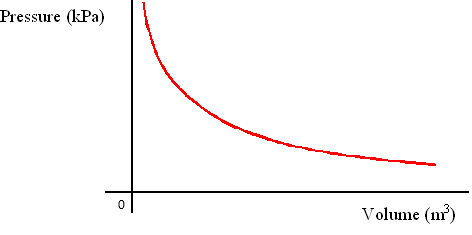Example of Boyle’s law :

When Air is filled into a balloon; the pressure of that air expend that balloon. If one end of the balloon is squeezed, making the volume smaller, the pressure inside increased, making the un-squeezed part of the balloon expand out.

Coulomb’s Law: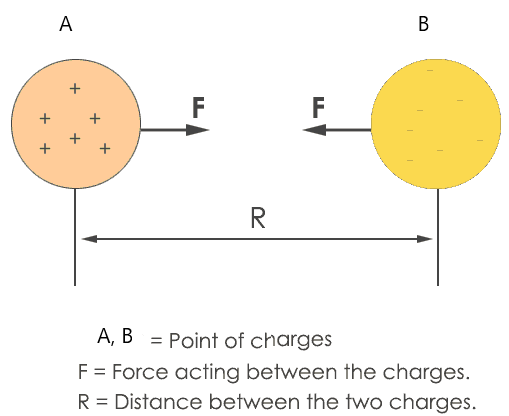This law states that the force of “attraction or repulsion” between two charged objects or bodies is directly proportional to the product of their charges and inversely proportional to the square of the distance between them.

• Coulomb’s law established by “Charles Augustin de Coulomb.”
• The SI unit of electric charge is “coulomb”.

For More:

If you like and think that General Science (Physics) topic on “Law’s of Physics (Mechanics)-भौतिकी के नियम” was helpful for you, Please comment us. Your comments/suggestions would be greatly appreciated. Thank you to be here. Regards – Team SukRaj Classes.